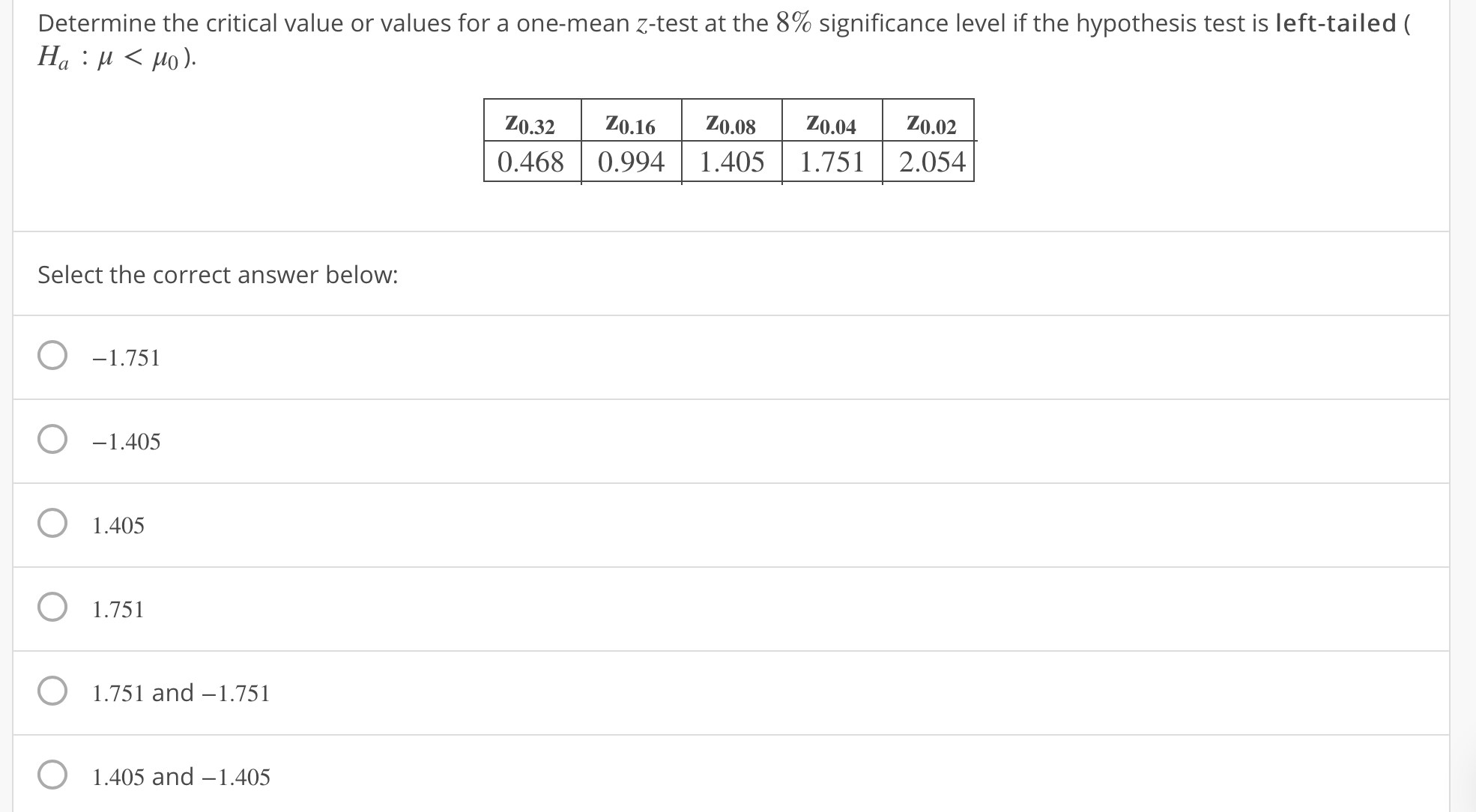Determine the critical value or values for a one-mean z-test at the 8% significance level if the hypothesis test is left-tailed (0.32 0.16 .8 0.040.020.468 0.994| 1.405 1.7512.054Select the correct answer below:O-1.751O -1.405O 1.405O1.751O 1.751 and -1.751O1.405 and -1.405

Questionhelp_outlineImage TranscriptioncloseDetermine the critical value or values for a one-mean z-test at the 8% significance level if the hypothesis test is left-tailed ( 0.32 0.16 .8 0.040.02 0.468 0.994| 1.405 1.7512.054 Select the correct answer below: O-1.751 O -1.405 O 1.405 O1.751 O 1.751 and -1.751 O 1.405 and -1.405 fullscreen
Step 1

Given one mean test at 8% significance level is carried out.

The hypothesis test is left tailed

Ho : µ < µo

From the gi...

Want to see the full answer?

See Solution

Want to see this answer and more?

Our solutions are written by experts, many with advanced degrees, and available 24/7

See Solution
Tagged in

Hypothesis Testing